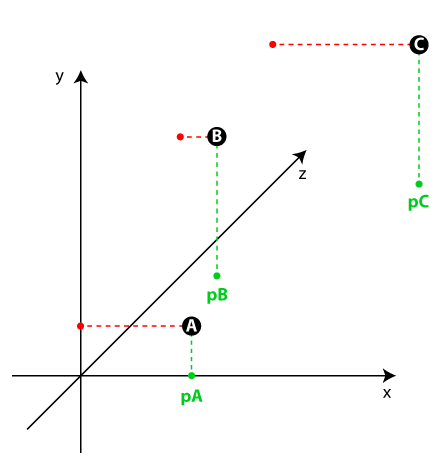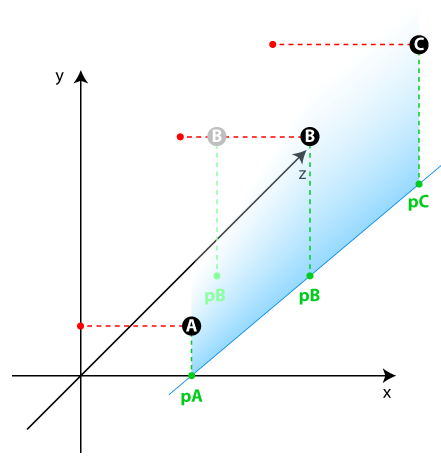# Position 3 points in space, on a same vertical plane (pure maths problem)

My problem is not specific to Unity, it’s purely mathematics. It has to do with projections, vectors, barycentric stuff and other line equations.

So I have 3 points, A, B and C, which are not aligned in space.

pA, pB and PC are their respective vertical projections on the « floor » (the xz plane). As they are also not aligned, they form a triangle on an horizontal plane.I need to move my B point on the x axis only, so that it belongs to the same vertical plane as A and C.

As a consequence, pA, pB and PC should now be aligned.I’m sure there are plenty of ways to do that, such as calculating pB’s coordinates with line equations, but I’m not sure what would be the right and the simpliest way.

Thanx in advance to all mathematician heroesTry something like this:

Calculate vector AC:

``````Vector3 AC = C - A;
``````

Project vector AC onto the XZ plane by setting the Y component to 0:

``````Vector3 AC_proj = new Vector3(AC.x, 0, AC.z);
``````

Find the intersection point between the XZ projection of AC and the X-axis. To do this, you can calculate the ratio of the Z components of A and AC_proj, and then use this ratio to interpolate the X components:

``````float ratio = (B - A).z / AC_proj.z;
float xIntersection = A.x + ratio * AC_proj.x;
``````

Set the X-coordinate of point B to the intersection point found in the previous step:

``````Vector3 newB = new Vector3(xIntersection, B.y, B.z);
``````

Now, point B is in the same vertical plane as A and C, and the projections pA, pB, and pC will be aligned on the XZ plane.

Let me know if that works.